Watch: How Luxury Mechanical Calculator Works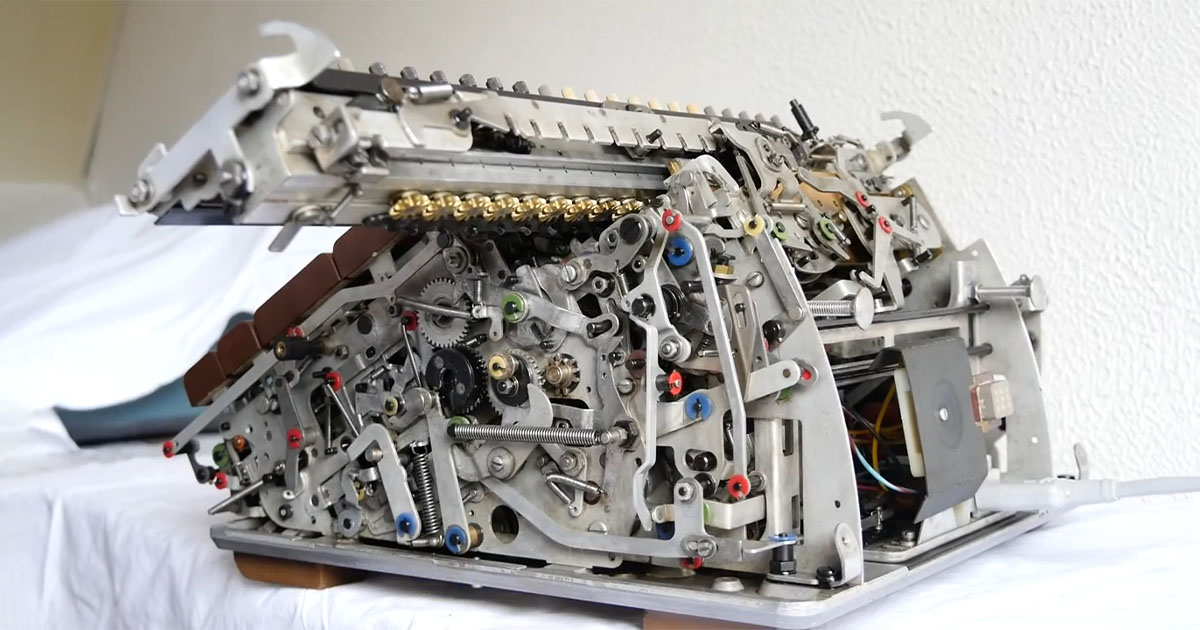Source: Friden SBT10 / YouTube

The mechanical calculator, also known as an arithmetic calculator, is the mechanical equipment utilized to carry out the basic operations of math. The first mechanical calculator, known as Pascal's calculator, was created in the 17th century, and exactly in 1642 by the French mathematician and physicist Blaise Pascal.

## Brief History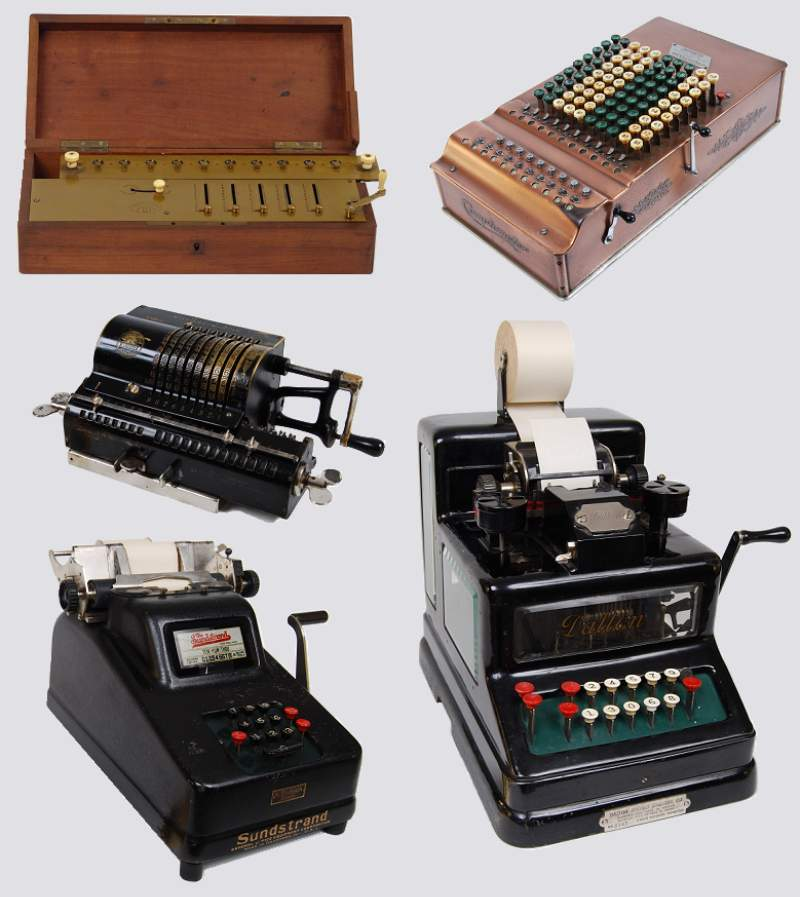Source: Ezrdr / wikicommons

The earliest mechanical calculators were proposed by polymaths and encyclopedic scientists, including Leonardo da Vinci, Wilhelm Schickard, Blaise Pascal, and Gottfried Leibniz up to the end of the 17th century.

The history of mechanical calculators began in the 17th century with the introduction of Pascal's calculator. Arithmetic tools were also created in the 17th century. Arithmometers dominated over and limited the usage of mechanical calculators until the mid-19th century introduction.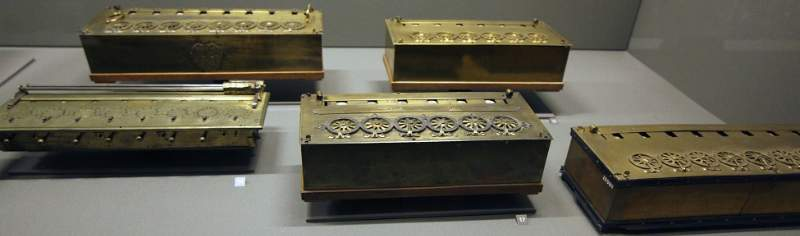Source: Edal Anton Lefterov / wikicommons

Blaise Pascal created a mechanical calculator in 1642. After three years and 50 prototypes, he unveiled his calculator. In the ten years that followed, he built twenty more. Those devices immediately add, subtract, multiply, and divide two integers.

## How it works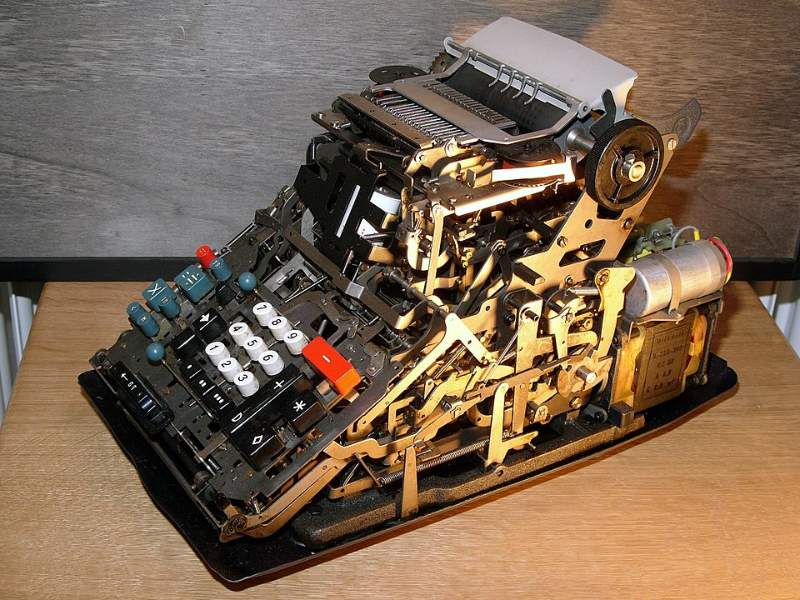Source: Hannes Grobe / wikicommons

Wheels and an abacus-like arrangement allow the user to input data. Single-toothed cogs would fail when the structure rolled over from 999 to 1,000 because of the structure's mechanical vulnerability. This may explain why Blaise Pascal was given credit for creating the mechanical calculator some 20 years later.

The most difficult component of making a mechanical calculator was calculating how to move gear in relation to the quantity being added. Stylus/slide adders have a straightforward answer to this problem.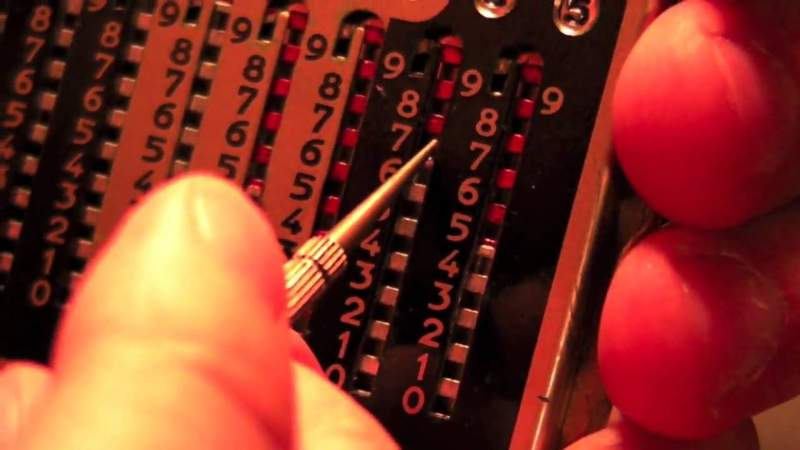Source: ThePaulClip / YouTube

In order to change the wheel or slide, the user simply puts the stylus into the appropriate hole. Because the user had no way of knowing if they had entered the proper number, this was a bad idea in the first place. The same number would have to be "dialed in" again when completing multiplication and division, making it difficult to remember.

## Conclusion

There had been a gradual shift away from mechanical calculators to electronic calculators in the early 1970s. They may appear outdated to us now, but they were incredible tools that allowed us to do impressive things with the technology we had at the time.

Watch the following video that shows how a mechanical calculator works to divide 355 by 113, which is the approximation of pi (π):